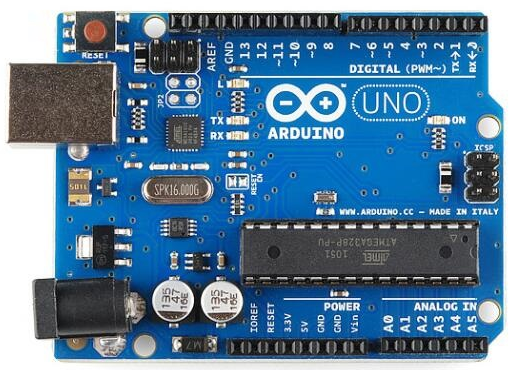# I want to use the arduino board to detect the distance in three directions at once

## Recommended Posts

Hello all.
As the question, I want to use the arduino board to detect the distance in three directions at once.the code compiled, uploaded are no problem, but can only return the data on the left, the middle and right are returned 0 value, I will be the left sensor code blocked can only return the data of the middle sensor, the right is still 0 value, the left and middle are blocked can return the data on the right , that is, the three ultrasonic sensors can That is, the three sensors can work separately, but can not return data together. That you have experience in this area to share, the code is as follows.

const int left_Trig = 1;
const int left_Echo = 2;
const int center_Trig = 3;
const int center_Echo = 4;
const int right_Trig = 5;
const int right_Echo = 6;
float a;
float b;
float c;
void setup()
{
Serial.begin(9600);
pinMode(left_Trig, OUTPUT);
pinMode(center_Trig, OUTPUT);
pinMode(right_Trig, OUTPUT);
pinMode(left_Echo, INPUT);
pinMode(center_Echo, INPUT);
pinMode(right_Echo, INPUT);
Serial.println("Ultrasonic sensor:");
}
void loop()
{

digitalWrite(left_Trig, LOW);
delayMicroseconds(2);
digitalWrite(center_Trig, LOW);
delayMicroseconds(2);
digitalWrite(right_Trig, LOW);
delayMicroseconds(2);
digitalWrite(left_Trig, HIGH);
delayMicroseconds(10);
digitalWrite(center_Trig, HIGH);
delayMicroseconds(10);
digitalWrite(right_Trig, HIGH);
delayMicroseconds(10);
digitalWrite(left_Trig, LOW);
digitalWrite(center_Trig, LOW);
digitalWrite(right_Trig, LOW);
a=pulseIn(left_Echo, HIGH) / 58.00;
Serial.println(a);
b=pulseIn(center_Echo, HIGH) / 58.00;
Serial.println(b);
c=pulseIn(right_Echo, HIGH) / 58.00;
Serial.println(c);
a = (int(a* 100.0)) / 100.0;
b = (int(b* 100.0)) / 100.0;
c = (int(c* 100.0)) / 100.0;
Serial.println(a);
Serial.println(b);
Serial.println(c);
Serial.println();
delay(10）;
}

## Donate your old hardware to community. Start a giveaway Raffle!

Not that I have experience with these sensors, but looks like the general flow is this:

• hit the sensor with a pulse (low/high)
• count cycles until a response (pulseIn)

As it's a microcontroller, pulseIn will probably not return until there is a response. The second call to pulseIn() probably starts counting after you're done printing the value from the first. Considering your output pulses happen within 2-10 ms of each other, I bet this happens:

```digitalWrite(center_Trig, LOW);
digitalWrite(right_Trig, LOW);
a=pulseIn(left_Echo, HIGH) / 58.00;
Serial.println(a);  // <- 2nd, 3rd echos arrive here
b=pulseIn(center_Echo, HIGH) / 58.00; // <- timeout
Serial.println(b);
c=pulseIn(right_Echo, HIGH) / 58.00; // <- timeout
Serial.println(c);```

Maybe pulse the second sensor after you're done reading the return from the first? Then the third when the second is done?

##### Share on other sites

The original code with commented problems:

```const int left_Trig = 1;
const int left_Echo = 2;
const int center_Trig = 3;
const int center_Echo = 4;
const int right_Trig = 5;
const int right_Echo = 6;
float a;
float b;
float c;
void setup()
{
Serial.begin(9600);
pinMode(left_Trig, OUTPUT);
pinMode(center_Trig, OUTPUT);
pinMode(right_Trig, OUTPUT);
pinMode(left_Echo, INPUT);
pinMode(center_Echo, INPUT);
pinMode(right_Echo, INPUT);
Serial.println("Ultrasonic sensor:");
}
void loop()
{

digitalWrite(left_Trig, LOW);
delayMicroseconds(2);
digitalWrite(center_Trig, LOW);
delayMicroseconds(2);
digitalWrite(right_Trig, LOW);
delayMicroseconds(2); // Why the delay when turning them all off? Why not just turn all low at the same time?

digitalWrite(left_Trig, HIGH);
delayMicroseconds(10);
digitalWrite(center_Trig, HIGH);
delayMicroseconds(10);
digitalWrite(right_Trig, HIGH);
delayMicroseconds(10); // Delay after turning each one on, guarantees at this point the left one was on for 30µs already, the center for 20µs and the right one for 10µs that cant be right?
// maybe again turn them all on, then wait 10µs?

digitalWrite(left_Trig, LOW);
digitalWrite(center_Trig, LOW);
digitalWrite(right_Trig, LOW); // this seems ok

a = pulseIn(left_Echo, HIGH) / 58.00;
Serial.println(a);
b = pulseIn(center_Echo, HIGH) / 58.00;
Serial.println(b);
c = pulseIn(right_Echo, HIGH) / 58.00;
Serial.println(c);

// this has problems because as tparys assumed pulseIn blocks. So the first pulseIn waits for the first echo, but then the second and so on might have been already missed!

a = (int(a * 100.0)) / 100.0;
b = (int(b * 100.0)) / 100.0;
c = (int(c * 100.0)) / 100.0;

// not sure what you are trying to do here? Multiply by 100 then divide by 100 just gives 1. So might just scrap it?

Serial.println(a);
Serial.println(b);
Serial.println(c);
Serial.println();
delay(10）;
}```

Improved code, using one function to get rid of duplication and read one sensor after the other:

```const int left_Trig = 1;
const int left_Echo = 2;

const int center_Trig = 3;
const int center_Echo = 4;

const int right_Trig = 5;
const int right_Echo = 6;

float a;
float b;
float c;

void setup()
{
Serial.begin(9600);
pinMode(left_Trig, OUTPUT);
pinMode(center_Trig, OUTPUT);
pinMode(right_Trig, OUTPUT);

pinMode(left_Echo, INPUT);
pinMode(center_Echo, INPUT);
pinMode(right_Echo, INPUT);

Serial.println("Ultrasonic sensor:");
}
void loop()
{

a = getEchoTime(left_Trig); // use a funtion here to get rid of the duplicate code
b = getEchoTime(center_Trig);
c = getEchoTime(right_Trig);

Serial.println(a);
Serial.println(b);
Serial.println(c);
Serial.println();

delay(10）;
}

float getEchoTime(int channel) {
digitalWrite(channel, LOW);
delayMicroseconds(2);  // turn channel of, and wait

digitalWrite(channel, HIGH);
delayMicroseconds(10); // turn channel on, then wait 10µs

digitalWrite(channel, LOW); // turn off, total pulse time 10µs

return pulseIn(channel + 1, HIGH) / 58.00; // use +1 here, as the echo channel seems to be trigger channel + 1
}```

An even better way would be to use interrupts (either external or pinchange) as this would allow you to fire the pulse and then do something else while waiting for the echo. I'm sure there are plenty of tutorials out there on how to read ultrasonic sensors in arduino without blocking.

## Join the conversation

You can post now and register later. If you have an account, sign in now to post with your account.
Note: Your post will require moderator approval before it will be visible.×   Pasted as rich text.   Restore formatting

Only 75 emoji are allowed.